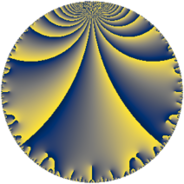# Properties

 Label 49.6.cLevel $49$ Weight $6$ Character orbit 49.c Rep. character $\chi_{49}(18,\cdot)$ Character field $\Q(\zeta_{3})$ Dimension $30$ Newform subspaces $8$ Sturm bound $28$ Trace bound $3$

# Related objects

## Defining parameters

 Level: $$N$$ $$=$$ $$49 = 7^{2}$$ Weight: $$k$$ $$=$$ $$6$$ Character orbit: $$[\chi]$$ $$=$$ 49.c (of order $$3$$ and degree $$2$$) Character conductor: $$\operatorname{cond}(\chi)$$ $$=$$ $$7$$ Character field: $$\Q(\zeta_{3})$$ Newform subspaces: $$8$$ Sturm bound: $$28$$ Trace bound: $$3$$ Distinguishing $$T_p$$: $$2$$, $$3$$

## Dimensions

The following table gives the dimensions of various subspaces of $$M_{6}(49, [\chi])$$.

Total New Old
Modular forms 54 38 16
Cusp forms 38 30 8
Eisenstein series 16 8 8

## Trace form

 $$30 q + 3 q^{2} - 8 q^{3} - 201 q^{4} - 38 q^{5} + 164 q^{6} - 90 q^{8} - 1381 q^{9} + O(q^{10})$$ $$30 q + 3 q^{2} - 8 q^{3} - 201 q^{4} - 38 q^{5} + 164 q^{6} - 90 q^{8} - 1381 q^{9} - 778 q^{10} + 916 q^{11} - 196 q^{12} + 1848 q^{13} - 2408 q^{15} - 4805 q^{16} - 2346 q^{17} + 1945 q^{18} - 360 q^{19} + 3416 q^{20} - 4388 q^{22} + 1284 q^{23} + 1392 q^{24} - 39 q^{25} + 1148 q^{26} - 5744 q^{27} + 16748 q^{29} + 5838 q^{30} + 3548 q^{31} - 16187 q^{32} - 3398 q^{33} - 14844 q^{34} - 3046 q^{36} + 40596 q^{37} + 20138 q^{38} + 6776 q^{39} + 15936 q^{40} - 7000 q^{41} + 65048 q^{43} + 13092 q^{44} + 1300 q^{45} - 5826 q^{46} - 22956 q^{47} - 22432 q^{48} - 194078 q^{50} + 11872 q^{51} - 1400 q^{52} + 23568 q^{53} + 32546 q^{54} + 50152 q^{55} + 204876 q^{57} - 141954 q^{58} - 65808 q^{59} + 67172 q^{60} - 42486 q^{61} + 98724 q^{62} - 129766 q^{64} - 106092 q^{65} - 25894 q^{66} - 34252 q^{67} + 5460 q^{68} - 10308 q^{69} - 18560 q^{71} - 35379 q^{72} - 50506 q^{73} - 217828 q^{74} - 35608 q^{75} - 77672 q^{76} - 154392 q^{78} - 55332 q^{79} + 68816 q^{80} + 132905 q^{81} + 67732 q^{82} + 208656 q^{83} + 628460 q^{85} + 458128 q^{86} + 80008 q^{87} + 229848 q^{88} - 26666 q^{89} - 261304 q^{90} - 394200 q^{92} + 6034 q^{93} + 98034 q^{94} - 295316 q^{95} + 54880 q^{96} - 418264 q^{97} - 724888 q^{99} + O(q^{100})$$

## Decomposition of $$S_{6}^{\mathrm{new}}(49, [\chi])$$ into newform subspaces

Label Dim $A$ Field CM Traces $q$-expansion
$a_{2}$ $a_{3}$ $a_{5}$ $a_{7}$
49.6.c.a $2$ $7.859$ $$\Q(\sqrt{-3})$$ $$\Q(\sqrt{-7})$$ $$-11$$ $$0$$ $$0$$ $$0$$ $$q-11\zeta_{6}q^{2}+(-89+89\zeta_{6})q^{4}+627q^{8}+\cdots$$
49.6.c.b $2$ $7.859$ $$\Q(\sqrt{-3})$$ None $$10$$ $$-14$$ $$-56$$ $$0$$ $$q+10\zeta_{6}q^{2}+(-14+14\zeta_{6})q^{3}+(-68+\cdots)q^{4}+\cdots$$
49.6.c.c $2$ $7.859$ $$\Q(\sqrt{-3})$$ None $$10$$ $$14$$ $$56$$ $$0$$ $$q+10\zeta_{6}q^{2}+(14-14\zeta_{6})q^{3}+(-68+\cdots)q^{4}+\cdots$$
49.6.c.d $4$ $7.859$ $$\Q(\sqrt{-3}, \sqrt{-19})$$ None $$-9$$ $$-6$$ $$-18$$ $$0$$ $$q+(5\beta _{1}+\beta _{2}-\beta _{3})q^{2}+(-6-6\beta _{1}+\cdots)q^{3}+\cdots$$
49.6.c.e $4$ $7.859$ $$\Q(\sqrt{-3}, \sqrt{-19})$$ None $$-9$$ $$6$$ $$18$$ $$0$$ $$q+(5\beta _{1}+\beta _{2}-\beta _{3})q^{2}+(6+6\beta _{1}-6\beta _{3})q^{3}+\cdots$$
49.6.c.f $4$ $7.859$ $$\Q(\sqrt{-3}, \sqrt{37})$$ None $$-2$$ $$-8$$ $$-38$$ $$0$$ $$q+(-\beta _{1}-\beta _{2})q^{2}+(-4+4\beta _{1}+\beta _{2}+\cdots)q^{3}+\cdots$$
49.6.c.g $4$ $7.859$ $$\Q(\sqrt{-3}, \sqrt{-13})$$ None $$4$$ $$0$$ $$0$$ $$0$$ $$q+(2-2\beta _{1})q^{2}+\beta _{2}q^{3}+28\beta _{1}q^{4}+\cdots$$
49.6.c.h $8$ $7.859$ 8.0.$$\cdots$$.19 None $$10$$ $$0$$ $$0$$ $$0$$ $$q+(3-2\beta _{1}-\beta _{3}-\beta _{6})q^{2}+(-2\beta _{2}+\cdots)q^{3}+\cdots$$

## Decomposition of $$S_{6}^{\mathrm{old}}(49, [\chi])$$ into lower level spaces

$$S_{6}^{\mathrm{old}}(49, [\chi]) \cong$$ $$S_{6}^{\mathrm{new}}(7, [\chi])$$$$^{\oplus 2}$$